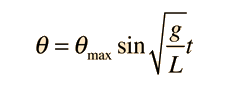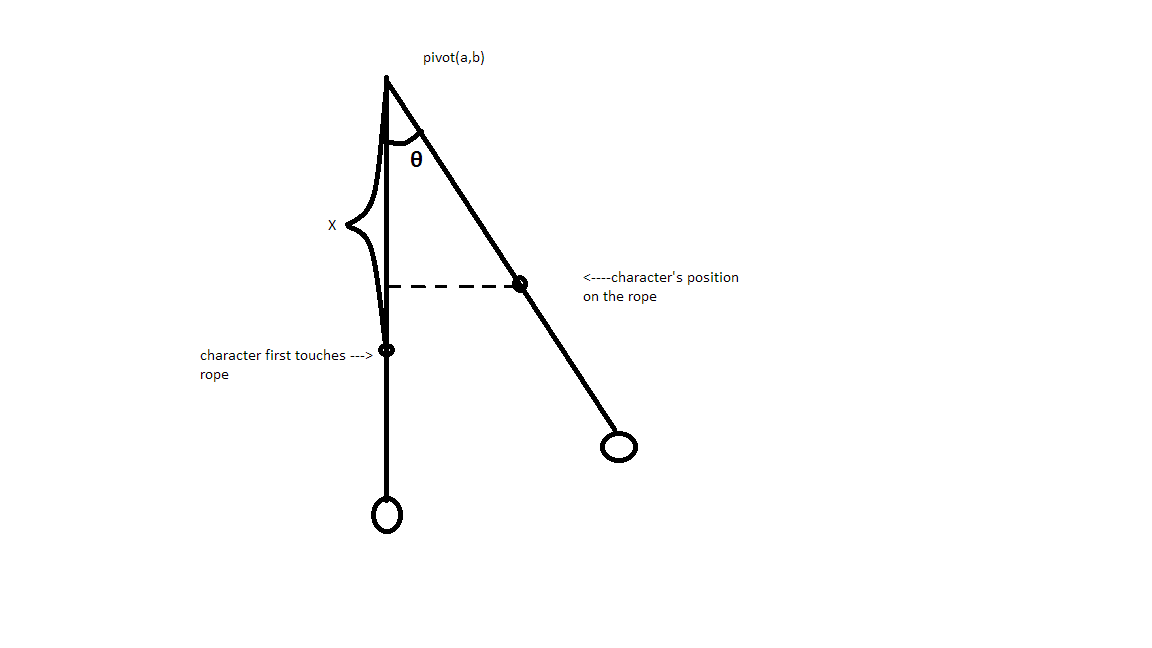# Rope Swing

## Tutorials

Simple demo with my tutorial:

In your character code, you only need to add a variable ifOnRope to see if the character is attached to rope; also, you need to modify your jump functin so that if you are on the rope, you jump and will set ifOnRope to false and leave the Rope:

## MyCharacter.cpp

```
void AMyCharacter::SetupPlayerInputComponent(class UInputComponent* InputComponent)
{
Super::SetupPlayerInputComponent(InputComponent);
InputComponent->BindAxis("Forward", this,
&AMyCharacter::MoveForward);
InputComponent->BindAxis("Strafe", this, &AMyCharacter::MoveRight);
InputComponent->BindAction("Jump", IE_Pressed, this, &AMyCharacter::OnStartJump);
InputComponent->BindAction("Jump", IE_Released, this, &AMyCharacter::OnStopJump);
ifOnRope = false;
}
void AMyCharacter::OnStartJump()
{

bPressedJump = true;
if (ifOnRope)
ifOnRope = false;
}
void AMyCharacter::OnStopJump()
{
bPressedJump = false;
}
```

Then we need to add some variable to the rope actor: float rotateDegree; // The "Max" swing degree float TotalTime; //Time span since character attach to rope, this may be improved by using TimeSpan structure FVector actorFirstLocation; // The location when character proxs rope FVector Pivot; //rope's pivot We use the following pendulum equation to calculate swing:pendulum equation

For simplicity, when character touches the rope, we set the maximum theta from 0 to 40 degree and let "G/L" be a value making your pendulum's speed appropriate. In my computer, I make it to be 1/30 and I get a pendulum with a moderate speed. When character is attached to rope, we set the totalTime to zero, in every tick, we add deltaTime to TotalTime, more efficient way may involve the usage of TimeSpan structure. Now after adding this function, your pendulum can swing after your character touches the rope, full code will be introduced later, so don't be intimidated if you are kind of confused now. SetActorRotation(FRotator(rotateDegree*sin(rotateDegree*sin(TotalTime)/30), 0.0f, 0.0f)); Now, your pendulum swings. What we need to do is to let the character swing at the same tempo, too; What we know is the rotation degree of the rope at each frame:FRotator(rotateDegree*sin(rotateDegree*sin(TotalTime)/30) We also know the pivot location(a,b,c), as illustrated by the following picture:pendulum equation

In two dimensional, if pivot is (a,b). Then character's position on rope is: (a+x*sin(θ), b-x*cos(θ)), where x is the distance from character to pivot In my application, only z-axis, the height, and x-axis, the range of the swing change. So it is Pivot + (x*sin(θ),0,-x*cos(θ)) Now we can see the whole picture:

## Rope.h

```
UCLASS()
class MYPROJECT5_API AMyActor : public AActor
{
GENERATED_BODY()

public:
// Sets default values for this actor's properties
AMyActor(const FObjectInitializer& ObjectInitializer);
float rotateDegree; // The Max swing degree
float TotalTime;	//Time span since character attach to rope, this may be improved by using TimeSpan structure
FVector actorFirstLocation; // The location when character proxs rope
FVector Pivot;				//rope's pivot
AMyCharacter* actorAttached;//Our character
// Called when the game starts or when spawned

virtual void BeginPlay() override;
UBoxComponent* ProxBox;
UStaticMeshComponent* Mesh;
UFUNCTION(BlueprintNativeEvent, Category = Collision)
void Prox(AActor* OtherActor, UPrimitiveComponent* OtherComp, int32 OtherBodyIndex, bool bFromSweep, const FHitResult &SweepResult);
void Prox_Implementation(AActor* OtherActor, UPrimitiveComponent* OtherComp, int32 OtherBodyIndex, bool bFromSweep,
const FHitResult & SweepResult);
// Called every frame
virtual void Tick(float DeltaSeconds) override;
};
```

Prox function detect when it overlaps with other actors.

## Rope.cpp

```
AMyActor::AMyActor(const FObjectInitializer& ObjectInitializer)
: Super(ObjectInitializer)
{
// Set this actor to call Tick() every frame.  You can turn this off to improve performance if you don't need it.
PrimaryActorTick.bCanEverTick = true;
rotateDegree = 0.0f;
TotalTime = 0.0f;
Mesh = ObjectInitializer.CreateDefaultSubobject<UStaticMeshComponent>(this,
TEXT("Mesh"));
RootComponent = Mesh;
ProxBox = ObjectInitializer.CreateDefaultSubobject<UBoxComponent>(this,
TEXT("ProxBox"));
&AMyActor::Prox);
ProxBox->AttachTo(RootComponent);
actorAttached = NULL;
actorFirstLocation = FVector(0.0f, 0.0f, 0.0f);
Pivot = FVector(-110.0f, 0.0f, 260.0f); // my pivot, I know the location, yours should be different

}
void AMyActor::Prox_Implementation(AActor* OtherActor,
UPrimitiveComponent* OtherComp, int32 OtherBodyIndex, bool
bFromSweep, const FHitResult & SweepResult)
{

actorAttached = Cast<AMyCharacter>(OtherActor); // set actorAttached to our character if it detects rope overlaps with our character
if (actorAttached != NULL && (actorAttached->ifOnRope == false))
{
TotalTime = 0.0f;         //initilize total time
actorAttached->ifOnRope = true;
rotateDegree = 40;         //set the max swing degree to 40
actorFirstLocation = actorAttached->GetActorLocation(); //record the location of overlap
}

}

void AMyActor::Tick( float DeltaTime )
{
Super::Tick( DeltaTime );
TotalTime += DeltaTime;
float DistanceToPivot = (actorFirstLocation - Pivot).Size(); // Calculate the distance from character to pivot, it is a constant when character swings the rope
if (actorAttached != NULL)
{

if (actorAttached->ifOnRope){
//let the rope swing
SetActorRotation(FRotator(rotateDegree*sin(rotateDegree*sin(TotalTime)/30), 0.0f, 0.0f));

//character swing
actorAttached->SetActorLocation(Pivot + FVector(DistanceToPivot*sin(rotateDegree*sin(TotalTime)/30), 0.0f, -cos(rotateDegree*sin(TotalTime)/30)*DistanceToPivot)
);

}
else
{
// If character leaves the rope, we must let it stop
if (rotateDegree>=0)
rotateDegree -= 2;
SetActorRotation(FRotator(rotateDegree*sin(rotateDegree*sin(TotalTime) / 30), 0.0f, 0.0f));
}
}

}
== Conclusion ==
This is a simple implement of rope swing. Hope it is helpful.
```